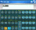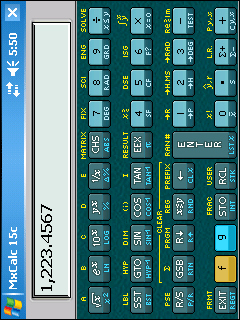Search
Software
Options for MxCalc 15c- PocketPC Calculator
MxCalc 15c- PocketPC CalculatorThe best Scientific & Engineering RPN calculator. Support for Complex numbers, Matrices, Statistical calculations, Trignometry, Integration, Solving equations & Basic arithmetic.
~ Looks as good as the HP-15c
~ Performs as good as the HP-15c
and more ..
A set of scientific & engineering functions, support for complex numbers, matrices & basic arithmetic.Esoteric features include transcendental functions &matrix operations..

Features

RPN Mode Calculator.
Programmable. KeyStroke programming- 499 Steps.
Hundreds of built-in functions.
Full Screen & Quick Stack Display.
Basic Mathematical calculations.
Complex number calculations.
Matrix operations.
Trigonometric functions.
Hyperbolic Trigonometry.
Combinations and Permutations.
Polar Rectangular conversions.
Time conversions.
Angle conversions.
Solver / Root finder.
Definite Integration
and more ....

and more ?.